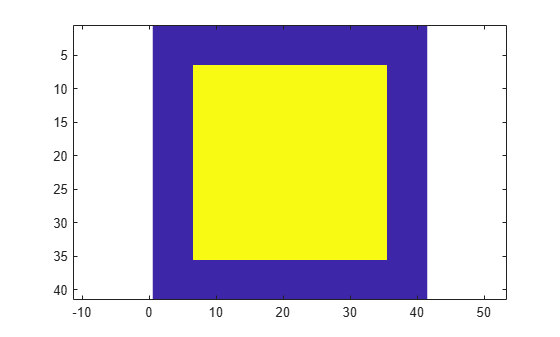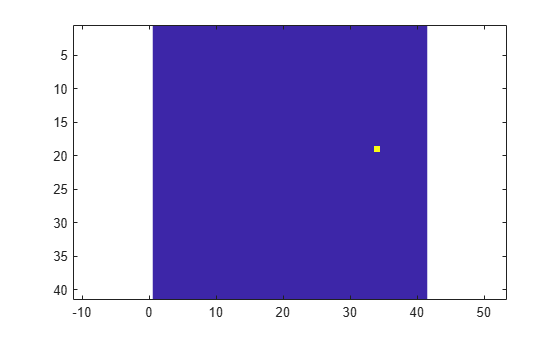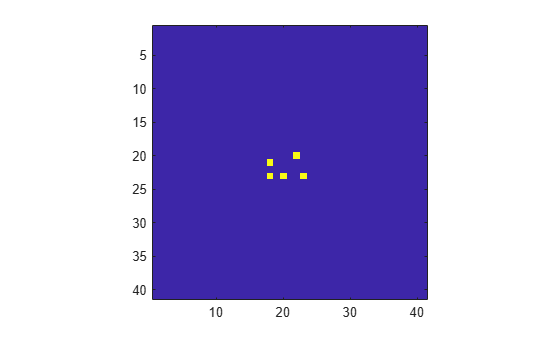Documentation

### This is machine translation

Mouseover text to see original. Click the button below to return to the English version of the page.

# phased.CFARDetector2D

Two-dimensional CFAR detector

## Description

The `phased.CFARDetector2D` System object™ implements a constant false-alarm rate detector (CFAR) for selected elements (called cells) of two-dimensional image data. A detection is declared when an image cell value exceeds a threshold. To maintain a constant false alarm-rate, the threshold is set to a multiple of the image noise power. The detector estimates noise power for a cell-under-test (CUT) from surrounding cells using one of three cell averaging methods, or an order statistics method. The cell-averaging methods are cell averaging (CA), greatest-of cell averaging (GOCA), or smallest-of cell averaging (SOCA).

For each test cell, the detector:

1. estimates the noise statistic from the cell values in the training band surrounding the CUT cell.

2. computes the threshold by multiplying the noise estimate by the threshold factor.

3. compares the CUT cell value to the threshold to determine whether a target is present or absent. If the value is greater than the threshold, a target is present.

To run the detector

1. Define and set up your 2-D CFAR detector. You can set the `phased.CFARDetector2D` System object properties when you create the object, or leave them set to their default values. See Construction. Some properties that you set at construction time can be changed later. These properties are tunable.

2. Find the detections by calling the `step` method. The output of this method depends on the properties of the `phased.CFARDetector2D` System object.

### Note

Alternatively, instead of using the `step` method to perform the operation defined by the System object, you can call the object with arguments, as if it were a function. For example, ```y = step(obj,x)``` and `y = obj(x)` perform equivalent operations.

## Construction

`detector = phased.CFARDetector2D` creates a 2-D CFAR detector System object, `detector`.

`detector = phased.CFARDetector2D(Name,Value)` creates a 2-D CFAR System object, `detector`, with each specified property `Name` set to the specified `Value`. You can specify additional name-value pair arguments in any order as (`Name1`,`Value1`,...,`NameN`,`ValueN`).

## Properties

expand all

Two-dimensional CFAR averaging method, specified as `'CA'`, `'GOCA'`, `'SOCA'`, or `'OS'`. For `'CA'`, `'GOCA'`, `'SOCA'`, the noise power is the sample mean derived from the training band. For `'OS'`, the noise power is the kth cell value obtained from numerically ordering all training cell values. Set k using the `Rank` property.

Averaging MethodDescription
`CA` — Cell-averaging algorithm

Computes the sample mean of all training cells surrounding the CUT cell.

`GOCA` — Greatest-of cell-averaging algorithm

Splits the 2-D training window surrounding the CUT cell into left and right halves. Then, the algorithm computes the sample mean for each half and selects the largest mean.

`SOCA` — Smallest-of cell-averaging algorithm

Splits the 2-D training window surrounding the CUT cell into left and right halves. Then, the algorithm computes the sample mean for each half and selects the smallest mean.

`OS` — Order statistic algorithm

Sorts training cells in ascending order of numeric values. Then the algorithm selects the kth value from the list. k is the rank specified by the `Rank` parameter.

Example: `'OS'`

Data Types: `char`

The number of rows and columns of the guard band cells on each side of the CUT cell, specified as nonnegative integers. The first element specifies the guard band size along the row dimension. The second element specifies the guard band size along the column dimension. Specifying this property as a single integer is equivalent to specifying a guard band with the same value for both dimensions. For example, a value of `[1 1]`, indicates that there is a one guard-cell-wide region surrounding each CUT cell. A value of zero indicates there are no guard cells.

Example: `[2 3]`

Data Types: `single` | `double`

The number of rows and columns of the training band cells on each side of the CUT cell, specified as a positive integer or a 1-by-2 matrix of positive integers. The first element specifies the training band size along the row dimension. The second element specifies the training band size along the column dimension. Specifying this property as a scalar is equivalent to specifying a training band with the same value for both dimensions. For example, a value of `[1 1]` indicates a 1 training-cell-wide region surrounding the CUT cell.

Example: `[-30:0.1:30]`

Data Types: `single` | `double`

Rank of the order statistic used in the 2-D CFAR algorithm, specified as a positive integer. The value of this property must lie between 1 and Ntrain, where Ntrain is the number of training cells. A value of 1 selects the smallest value in the training region.

Example: `5`

#### Dependencies

To enable this property, set the `Method` property to `'OS'`.

Data Types: `single` | `double`

Threshold factor method, specified as `'Auto'`, ```'Input port'```, or `'Custom'`.

When you set the `ThresholdFactor` property to `'Auto'`, the threshold factor is calculated from the desired probability of false alarm set in the `ProbabilityFalseAlarm` property. The calculation assumes that each independent signal in the input is a single pulse coming out of a square law detector with no pulse integration. In addition, the noise is assumed to be white Gaussian.

When you set the `ThresholdFactor` property to `'Input port'`, the threshold factor is obtained from an input argument of the `step` method.

When you set the `ThresholdFactor` property to `'Custom'`, the threshold factor is obtained from the value of the `CustomThresholdFactor` property.

Example: `'Custom'`

Data Types: `char`

Required probability of false alarm, specified as a real positive scalar between 0 and 1. The algorithm calculates the threshold factor from the required probability of false alarm.

Example: `0.001`

#### Dependencies

To enable this property, set the `ThresholdFactor` property to `'Auto'`.

Data Types: `single` | `double`

Custom threshold factor, specified as a real positive scalar. This property is tunable.

#### Dependencies

To enable this property, set the `ThresholdFactor` property to `'Custom'`.

Data Types: `single` | `double`

Format of detection results returned by the `step` method, specified as `'CUT result'` or ```'Detection index'```.

• When set to `'CUT result'`, the results are logical detection values (`1` or `0`) for each tested cell.

• When set to `'Detection index'`, the results form a vector or matrix containing the indices of tested cells that exceed a detection threshold. You can use this format as input to the `phased.RangeEstimator` and `phased.DopplerEstimator` System objects.

Data Types: `char`

Option to enable detection threshold output, specified as `false` or `true`. Setting this property to `true` returns the detection threshold via an output argument, `th`, of the `step` method.

Data Types: `logical`

Option to enable output of noise power, specified as `false` or `true`. Setting this property to `true` returns the noise power via the output argument, `noise`, of the `step` method.

Data Types: `logical`

Source of the number of detections, specified as `'Auto'` or `'Property'`. When you set this property to `'Auto'`, the number of detection indices reported is the total number of cells under test that have detections. If you set this property to `'Property'`, the number of reported detections is determined by the value of the `NumDetections` property.

#### Dependencies

To enable this property, set the `OutputFormat` property to `'Detection index'`.

Data Types: `char`

Maximum number of detection indices to report, specified as a positive integer.

Example:

#### Dependencies

To enable this property, set the `OutputFormat` property to `'Detection index'` and the `NumDetectionsSource` property to `'Property'`.

Data Types: `double`

## Methods

 reset Reset states of System object step Two-dimensional CFAR detection
Common to All System Objects
`release`

Allow System object property value changes

## Examples

expand all

This example shows how to set a 2-D CFAR threshold based upon a required probability of false alarm (pfa).

Note: You can replace each call to the function with the equivalent `step` syntax. For example, replace `myObject(x)` with `step(myObject,x)`.

Perform cell-averaging CFAR detection on a 41-by-41 matrix of cells containing Gaussian noise. Estimate the empirical pfa and compare it to the required pfa. To get a good estimate, perform this simulation on 1000 similar matrices. First, set a threshold using the required pfa. In this case, there are no targets and the pfa can be estimated from the number of cells that exceed the threshold. Assume that the data is processed through a square-law detector and that no pulse integration is performed. Use a training-cell band of 3 cells in width and 4 cells in height. Use a guard band of 3 cells in width and 2 cells in height to separate the cells under test (CUT) from the training cells. Specify a required pfa of 5.0e-4.

```p = 5e-4; rs = RandStream.create('mt19937ar','Seed',5); N = 41; ntrials = 1000; detector = phased.CFARDetector2D('TrainingBandSize',[4,3], ... 'ThresholdFactor','Auto','GuardBandSize',[2,3], ... 'ProbabilityFalseAlarm',p,'Method','SOCA','ThresholdOutputPort',true);```

Create a 41-by-41 image containing random complex data. Then, square the data to simulate a square-law detector.

```x = 2/sqrt(2)*(randn(rs,N,N,ntrials) + 1i*randn(rs,N,N,ntrials)); x2 = abs(x).^2;```

Process all the cells in each image. To do this, find the row and column of each CUT cell whose training region falls entirely within each image.

```Ngc = detector.GuardBandSize(2); Ngr = detector.GuardBandSize(1); Ntc = detector.TrainingBandSize(2); Ntr = detector.TrainingBandSize(1); cutidx = []; colstart = Ntc + Ngc + 1; colend = N - ( Ntc + Ngc); rowstart = Ntr + Ngr + 1; rowend = N - ( Ntr + Ngr); for m = colstart:colend for n = rowstart:rowend cutidx = [cutidx,[n;m]]; end end ncutcells = size(cutidx,2);```

Display the CUT cells.

```cutimage = zeros(N,N); for k = 1:ncutcells cutimage(cutidx(1,k),cutidx(2,k)) = 1; end imagesc(cutimage) axis equal```Perform the detection on all CUT cells. Return the detection classification and the threshold used to classify the cell.

`[dets,th] = detector(x2,cutidx);`

Find and display an image with a false alarm for illustration.

```di = []; for k = 1:ntrials d = dets(:,k); if (any(d) > 0) di = [di,k]; end end idx = di(1); detimg = zeros(N,N); for k = 1:ncutcells detimg(cutidx(1,k),cutidx(2,k)) = dets(k,idx); end imagesc(detimg) axis equal```Compute the empirical pfa.

`pfa = sum(dets(:))/ntrials/ncutcells`
```pfa = 4.5898e-04 ```

The empirical and specified pfa agree.

Display the average empirical threshold value over all images.

`mean(th(:))`
```ans = 31.7139 ```

Compute the theoretical threshold factor for the required pfa.

```threshfactor = npwgnthresh(p,1,'noncoherent'); threshfactor = 10^(threshfactor/10); disp(threshfactor)```
``` 7.6009 ```

The theoretical threshold factor multiplied by the noise variance should agree with the measured threshold.

```noisevar = mean(x2(:)); disp(threshfactor*noisevar);```
``` 30.4118 ```

The theoretical threshold and empirical threshold agree to within an acceptable difference.

Perform cell-averaging CFAR detection on a 41-by-41 matrix of cells containing five closely-spaced targets in Gaussian noise. Perform this detection on a simulation of 1000 images. Use two detectors with different guard band regions. Set the thresholds manually using the `Custom` threshold factor. Assume that the data is processed through a square law-detector and that no pulse integration is performed. Use a training cell band of 2 cells in width and 2 cells in height. For the first detector, use a guard band of 1 cell all around to separate the CUT cells from the training cells. For the second detector, use a guard band of 8 cells all around.

Note: This example runs only in R2016b or later. If you are using an earlier release, replace each call to the function with the equivalent `step` syntax. For example, replace `myObject(x)` with `step(myObject,x)`.

```p = 5e-4; rs = RandStream.create('mt19937ar','Seed',5); N = 41; ntrials = 1000;```

Create 1000 41-by-41 images of complex random noise with standard deviation of 1.

```s = 1; x = s/sqrt(2)*(randn(rs,N,N,ntrials) + 1i*randn(rs,N,N,ntrials));```

Set the target cells values to 1.5. Then, square the cell values.

```A = 1.5; x(23,20,:) = A; x(23,18,:) = A; x(23,23,:) = A; x(20,22,:) = A; x(21,18,:) = A; x2 = abs(x).^2;```

Display the target cells.

```xtgt = zeros(N,N); xtgt(23,20,:) = A; xtgt(23,18,:) = A; xtgt(23,23,:) = A; xtgt(20,22,:) = A; xtgt(21,18,:) = A; imagesc(xtgt) axis equal axis tight```Set the CUT cells to be the target cells.

```cutidx(1,1) = 23; cutidx(2,1) = 20; cutidx(1,2) = 23; cutidx(2,2) = 18; cutidx(1,3) = 23; cutidx(2,3) = 23; cutidx(1,4) = 20; cutidx(2,4) = 22; cutidx(1,5) = 21; cutidx(2,5) = 18;```

Perform the detection on all CUT cells using two CFAR 2-D detectors. The first detector has a small guard band region. The training region can include neighboring targets which can affect the computation of the noise power. The second detector has a larger guard band region, which precludes target cells from being used in the noise computation.

Create the two CFAR detectors.

```detector1 = phased.CFARDetector2D('TrainingBandSize',[2,2], ... 'GuardBandSize',[1,1],'ThresholdFactor','Custom','Method','CA', ... 'CustomThresholdFactor',2,'ThresholdOutputPort',true); detector2 = phased.CFARDetector2D('TrainingBandSize',[2,2], ... 'GuardBandSize',[8,8],'ThresholdFactor','Custom','Method','CA', ... 'CustomThresholdFactor',2,'ThresholdOutputPort',true);```

Return the detection classifications and the thresholds used to classify the cells. Then, compute the probabilities of detection.

```[dets1,th1] = detector1(x2,cutidx); ndets = numel(dets1(:)); pd1 = sum(dets1(:))/ndets```
```pd1 = 0.6416 ```
```[dets2,th2] = detector2(x2,cutidx); pd2 = sum(dets2(:))/ndets```
```pd2 = 0.9396 ```

The detector with the larger guard-band region has a higher pfa because the noise is more accurately estimated.

expand all

expand all

## References

 Mott, H. Antennas for Radar and Communications. New York: John Wiley & Sons, 1992.

 Richards, M. A. Fundamentals of Radar Signal Processing. New York: McGraw-Hill, 2005.

 Skolnik, M. Introduction to Radar Systems, 3rd Ed. New York: McGraw-Hill, 2001.